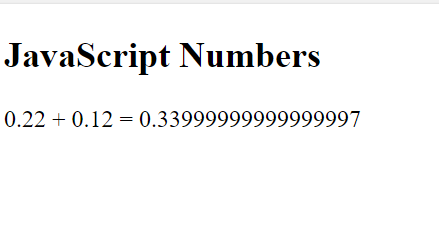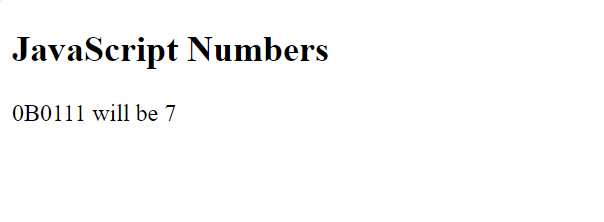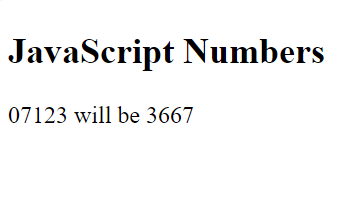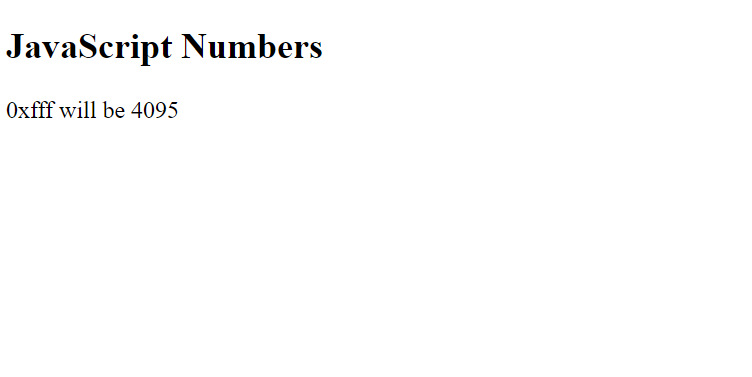# JavaScript | Numbers

JavaScript numbers are always stored as double-precision 64-bit binary format IEEE 754.

This format stores numbers in 64 bits,

• 0-51 bit stores value(fraction)
• 52-62 bit stores exponent
• 63 bit stores sign

The types of number literals You can use decimal, binary, octal, and hexadecimal.

• Decimal Numbers:
JavaScript Numbers does not have different types of numbers(ex: int, float, long, short) which other programming languages do. It has only one type of number and it can hold both with or without decimal values.

```Var a=33;
var b=3.3;
var x = 0562   //x will be 370(parsed as an octal number).```

If the number starts with 0 and the following number is smaller than 8. It will be parsed as an Octal Number.

Integers are accurate up to 15 digits:

```var a = 999999999999999;   // a will be 999999999999999
var b = 9999999999999999;     // b will be 10000000000000000```

Floating point is not 100% accurate. The maximum number of decimals is up to 17.

`var x = 0.22 + 0.12;   //x will be 0.33999999999999997`

Example-1:

 ` ` `<``html``> ` ` `  `<``body``> ` ` `  `    ``<``h2``> ` `      ``JavaScript Numbers ` `  `` ` ` `  `    ``<``p` `id``=``"num"``> ` ` `  `    ``<``script``> ` `        ``var x = 0.22 + 0.12; ` `        ``document.getElementById( ` `          ``"num").innerHTML =  ` `          ``"0.22 + 0.12 = " + x; ` `    `` ` ` `  ` ` ` `  ` `

Output:• Binary Numbers
They starts with 0b or 0B followed by 0’s and 1’s.

```var x = 0b11;        // x will be 3
var x = 0B0111;       // x will be 7```

Example-2:

 ` ` `<``html``> ` ` `  `<``body``> ` ` `  `    ``<``h2``> ` `      ``JavaScript Numbers ` `  `` ` ` `  `    ``<``p` `id``=``"num"``> ` ` `  `    ``<``script``> ` `        ``var x = 0 B0111; ` `        ``document.getElementById( ` `          ``"num").innerHTML =  ` `          ``"0B0111 will be " + x; ` `    `` ` ` `  ` ` ` `  ` `

Output:• Octal Numbers
They start with 0 followed by a number ranges from 0-7.if any number is used it will be taken as a decimal number.

```var x = 0111;      //x will be 73
var x = 07123;     //x will be 3667```

Example-3:

 ` ` `<``html``> ` ` `  `<``body``> ` ` `  `    ``<``h2``> ` `      ``JavaScript Numbers ` `  `` ` ` `  `    ``<``p` `id``=``"num"``> ` ` `  `    ``<``script``> ` `        ``var x = 07123; ` `        ``document.getElementById( ` `          ``"num").innerHTML =  ` `          ``"07123 will be " + x; ` `    `` ` ` `  ` ` ` `  ` `

Output:They start with 0x or 0X followed by any digit belonging (0123456789ABCDEF)

`var x = 0xfff;         //x will be 4095`

Example-2:

 ` ` `<``html``> ` ` `  `<``body``> ` ` `  `    ``<``h2``>JavaScript Numbers ` ` `  `    ``<``p` `id``=``"num"``> ` ` `  `    ``<``script``> ` `        ``var x = 0xfff; ` `        ``document.getElementById( ` `          ``"num").innerHTML =  ` `          ``"0xfff will be " + x; ` `    `` ` ` `  ` ` ` `  ` `

Output:• Exponentiation:
```var x = 2E5   // x will be 200000
var x = 34e3   // x will be 34000
var x = 23e-5 //x will be 0.00023 ```
• JavaScript uses the + operator for both addition and concatenation.
```var a = 20;
var b = 20;
var c = a + b;           // c will be 40 (a number)```
• Concatenation:
```var a = "10";
var b = "20";
var c = a + b;           // c will be 2020 (a string)```
• Numeric Strings
NaN is a reserved word indicating not a number.

``` var x= "12345" ;        // x will be a string(NaN)
var x = 67 ;            // x will be number```

My Personal Notes arrow_drop_upCheck out this Author's contributed articles.

If you like GeeksforGeeks and would like to contribute, you can also write an article using contribute.geeksforgeeks.org or mail your article to contribute@geeksforgeeks.org. See your article appearing on the GeeksforGeeks main page and help other Geeks.

Please Improve this article if you find anything incorrect by clicking on the "Improve Article" button below.

Article Tags :

Be the First to upvote.

Please write to us at contribute@geeksforgeeks.org to report any issue with the above content.